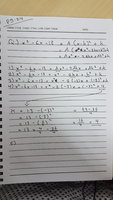# Completing the square question.

#### GhettoBurger

##### New member
I am currently going through a book called no bullshit guide to math and physics, and am puzzled by an exercise given early on in the book.

The question states x^2 − 6x + 13 = (x − 3)^2 + 4

In the chapter it goes on to state that any quadratic expression Ax^2 + Bx + C can be rewritten in the form A(x − h)^2 + k. Keeping this in mind I attempt to solve the question above.

I have done the equation as posted in the image below. But the final step which should give me the result (x − 3)^2 + 4 I am unable to reach. Could give me some guidance as to what I am doing incorrectly.#### Harry_the_cat

##### Senior Member
x^2 -6x -13 =x^2 -6x +(-3)^2 -(-3)^2 -13 = (x-3)^2 -9-13=(x-3)^2 - 22

#### MarkFL

##### Super Moderator
Staff member
To work the problem in the manner you have chosen (where the constant term on the left is +13 not -13), we have:

$$\displaystyle x^2-6x+13=Ax^2-2Ahx+Ah^2+k$$

Now, equating the coefficients of the squared terms, we find:

$$\displaystyle A=1$$

Looking at the linear terms, we find (where we let $$A=1$$):

$$\displaystyle -6=-2h\implies h=3$$

And finally the constant terms:

$$\displaystyle 13=9+k\implies k=4$$

Now, what I would choose to do here is write:

$$\displaystyle x^2-6x+13=x^2-6x+9+4=(x^2-2\cdot3\cdot x+3^2)+4=(x-3)^2+4$$

•GhettoBurger and topsquark

#### Harry_the_cat

##### Senior Member
Is it -13 or +13 in the OP? I took the first line of the handwritten work.

#### pka

##### Elite Member
I am currently going through a book called no bullshit guide to math and physics, and am puzzled by an exercise given early on in the book.

The question states x^2 − 6x + 13 = (x − 3)^2 + 4

In the chapter it goes on to state that any quadratic expression Ax^2 + Bx + C can be rewritten in the form A(x − h)^2 + k. Keeping this in mind I attempt to solve the question above.

I have done the equation as posted in the image below. But the final step which should give me the result (x − 3)^2 + 4 I am unable to reach. Could give me some guidance as to what I am doing incorrectly.

View attachment 12212
Do not complement things. It ain't hard.
\displaystyle \begin{align*}x^2-6x+13&=x^2-6x+9+4 \\&=(x-3)^2+4 \end{align*}

p.s. I did not see any of those other replies. So please cool the out rage.

Last edited:
•MarkFL

#### MarkFL

##### Super Moderator
Staff member
Is it -13 or +13 in the OP? I took the first line of the handwritten work.
I interpreted the post as having a typo in the handwritten work, as $$(x-3)^2+4$$ was stated as the desired result.

#### Harry_the_cat

##### Senior Member
Yeah I see now. You are right.

#### GhettoBurger

##### New member
Apologies everyone, MarkFL was correct there was a typo in my handwriting

But what I do not quite understand is how we went from this equation = (x^2 − 2 ⋅ 3 ⋅ x + 3^2) + 4 to this (x − 3) 2 + 4

Could you please clarify, before conintuing with any other problem I would like to fully understand this one.

Last edited:

#### MarkFL

##### Super Moderator
Staff member
Apologies everyone, MarkFL was correct there was a type in my handwriting

But what I do not quite understand is how we went from this equation = (x^2 − 2 ⋅ 3 ⋅ x + 3^2) + 4 to this (x − 3) 2 + 4

Could you please clarify, before conintuing with any other problem I would like to fully understand this one.
What we have to recognize here is the following:

$$\displaystyle (a-b)^2=a^2-2ab+b^2$$

Now, we should observe that:

$$\displaystyle x^2-2\cdot3\cdot x+3^2$$

fits that form, where $$a=x$$ and $$b=3$$. But, then you might ask, well, how did you know to break 13 up into the sum of 9 and 4? So, let's go back to the original expression:

$$\displaystyle x^2-6x+13$$

Now, let's look at the coefficient of the linear term which is $$-6$$. If we divide by 2 and square, we get:

$$\displaystyle \left(\frac{-6}{2}\right)^2=9$$

This tells us we need a 9 as part of the square we're trying to build, and so we break 13 into 9+4, giving us:

$$\displaystyle x^2-6x+9+4$$

And, we are assured the first 3 terms can be written as the square of a binomial.

Does that make sense?

#### GhettoBurger

##### New member
I think so, I get the general idea but I'll have to solve similar problems to be gain a certain amount of confidence that'll allow me to move on to the next subject matter with ease

I appreciate the help, thank you and thanks to the rest that contributed as well.

Heres to a long math journey ahead of me, with many more questions to come